Скачать презентацию Global Asset Allocation and Stock Selection Optimal Adjustment

618c15c2d7d3019c55f17b78a4fe5f73.ppt

• Количество слайдов: 10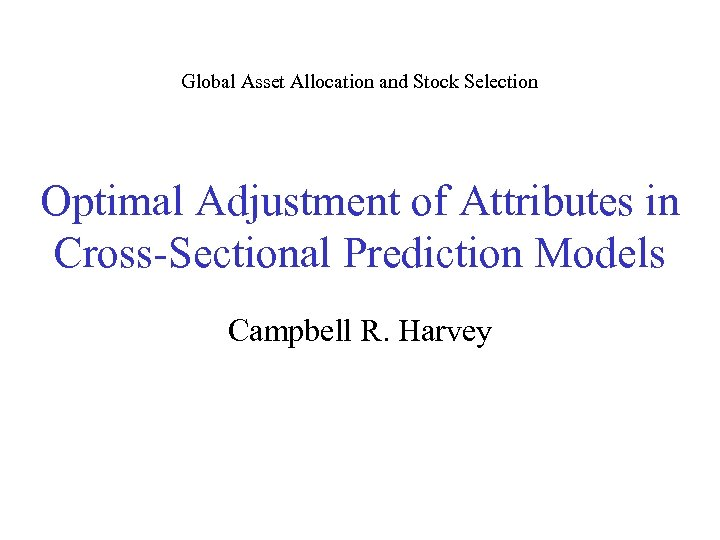Global Asset Allocation and Stock Selection Optimal Adjustment of Attributes in Cross-Sectional Prediction Models Campbell R. Harvey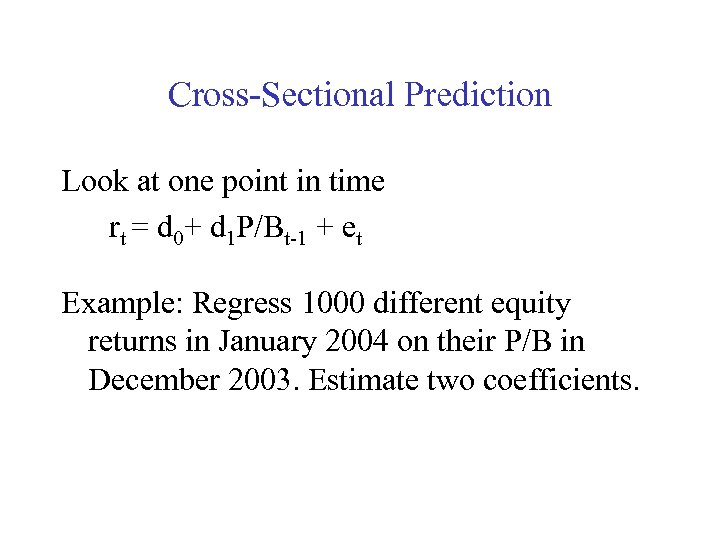Cross-Sectional Prediction Look at one point in time rt = d 0+ d 1 P/Bt-1 + et Example: Regress 1000 different equity returns in January 2004 on their P/B in December 2003. Estimate two coefficients.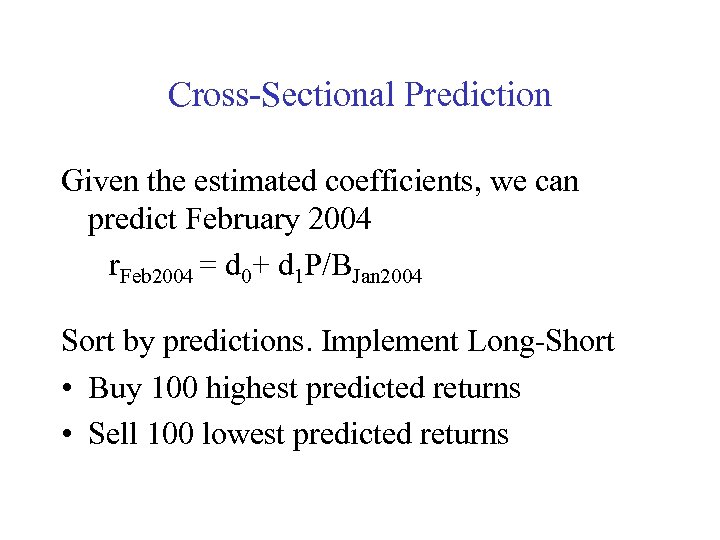Cross-Sectional Prediction Given the estimated coefficients, we can predict February 2004 r. Feb 2004 = d 0+ d 1 P/BJan 2004 Sort by predictions. Implement Long-Short • Buy 100 highest predicted returns • Sell 100 lowest predicted returns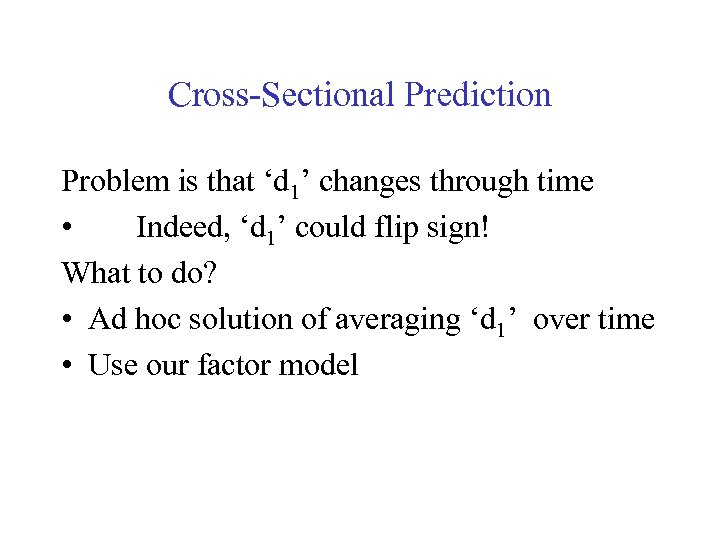Cross-Sectional Prediction Problem is that ‘d 1’ changes through time • Indeed, ‘d 1’ could flip sign! What to do? • Ad hoc solution of averaging ‘d 1’ over time • Use our factor model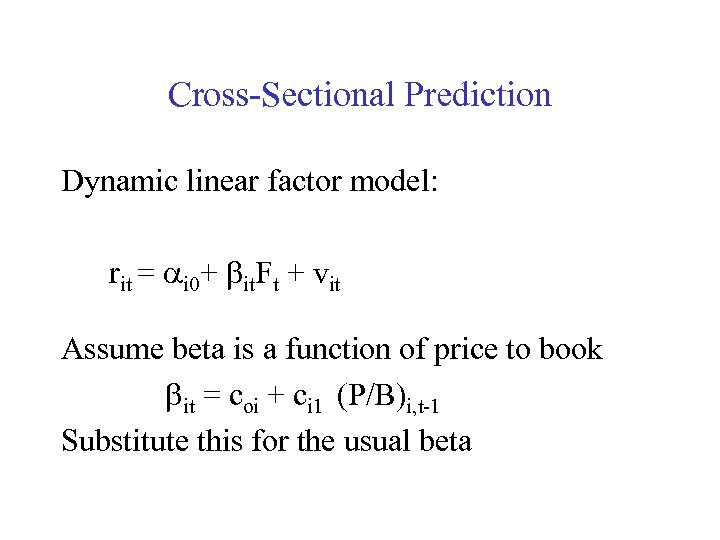Cross-Sectional Prediction Dynamic linear factor model: rit = ai 0+ bit. Ft + vit Assume beta is a function of price to book bit = coi + ci 1 (P/B)i, t-1 Substitute this for the usual beta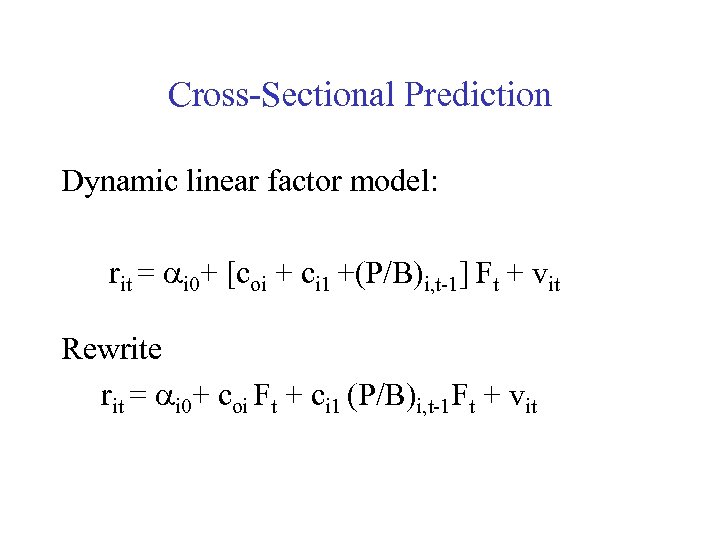Cross-Sectional Prediction Dynamic linear factor model: rit = ai 0+ [coi + ci 1 +(P/B)i, t-1] Ft + vit Rewrite rit = ai 0+ coi Ft + ci 1 (P/B)i, t-1 Ft + vit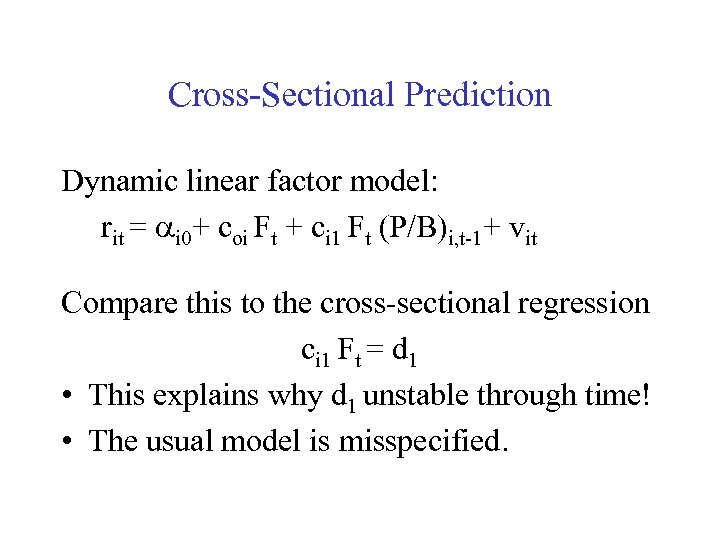Cross-Sectional Prediction Dynamic linear factor model: rit = ai 0+ coi Ft + ci 1 Ft (P/B)i, t-1+ vit Compare this to the cross-sectional regression ci 1 Ft = d 1 • This explains why d 1 unstable through time! • The usual model is misspecified.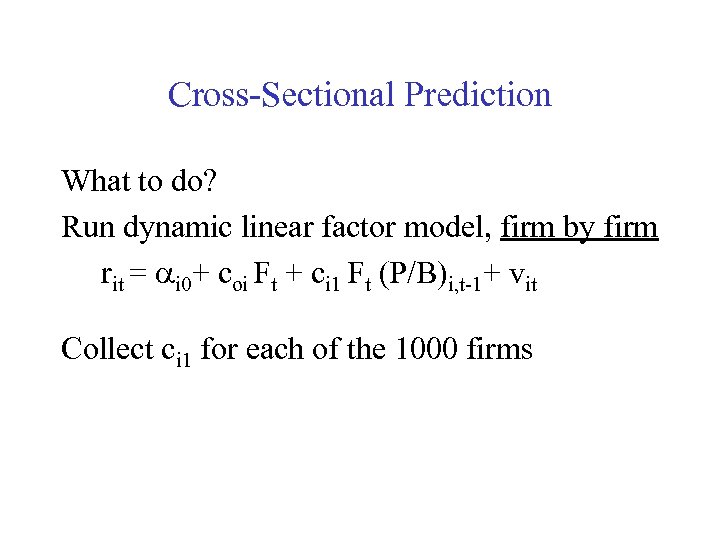Cross-Sectional Prediction What to do? Run dynamic linear factor model, firm by firm rit = ai 0+ coi Ft + ci 1 Ft (P/B)i, t-1+ vit Collect ci 1 for each of the 1000 firms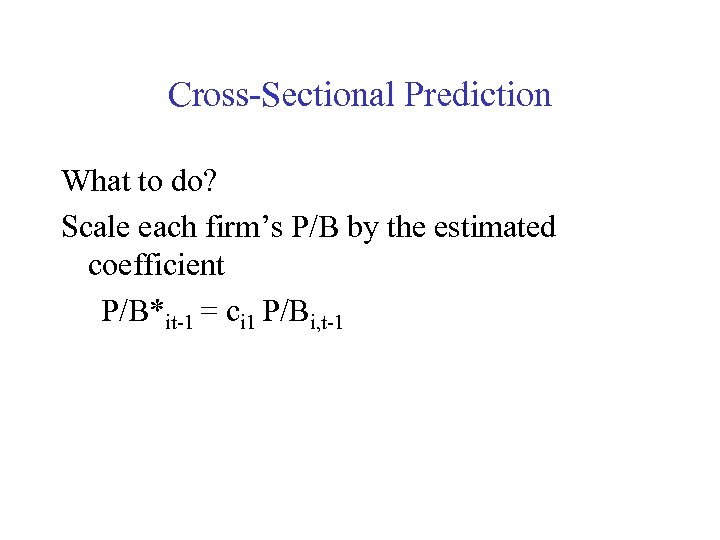Cross-Sectional Prediction What to do? Scale each firm’s P/B by the estimated coefficient P/B*it-1 = ci 1 P/Bi, t-1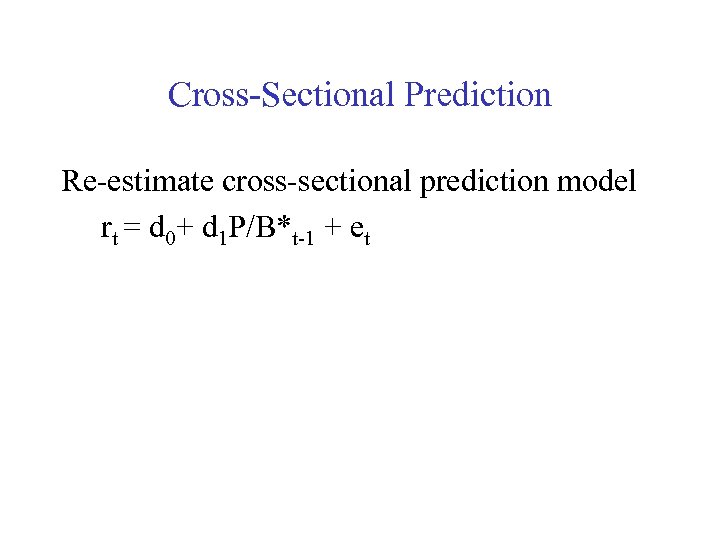Cross-Sectional Prediction Re-estimate cross-sectional prediction model rt = d 0+ d 1 P/B*t-1 + et